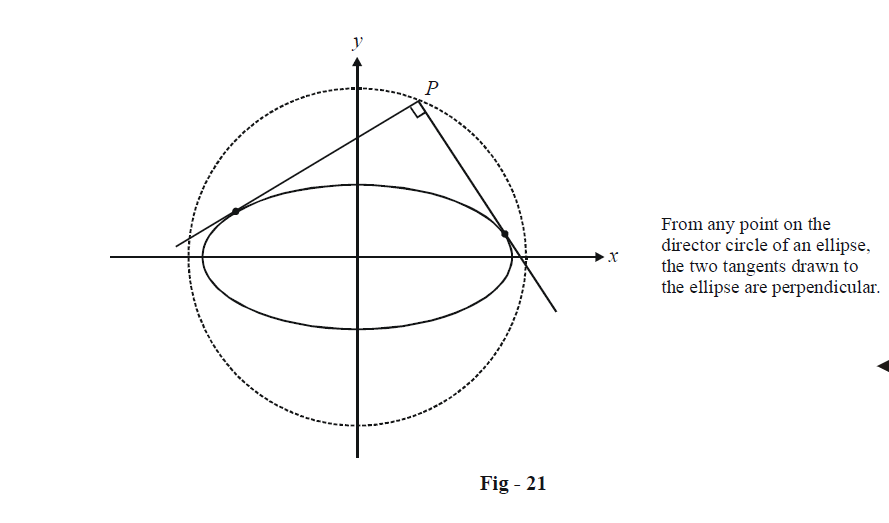# Examples On Tangents To Ellipses Set-1

Go back to  'Ellipse'

Example – 16

Tangents drawn at $$A({\theta _1})andB({\theta _2})$$ on the ellipse $$\frac{{{x^2}}}{{{a^2}}} + \frac{{{y^2}}}{{{b^2}}} = 1$$ intersect in P. Find the coordinates of P.

Solution: The equations of the tangents at A and B, using the parametric form for the tangent, are

\begin{align}&\frac{x}{a}\cos {\theta _1} + \frac{y}{b}\sin {\theta _1} = 1\qquad\qquad...\left( 1 \right)\\&\frac{x}{a}\cos {\theta _1} + \frac{y}{b}\sin {\theta _1} = 1\qquad\qquad...\left( 2 \right)\end{align}

$$(1) \times \cos {\theta _2} - (2) \times \cos {\theta _1}gives$$

\begin{align}&\frac{y}{b}\sin ({\theta _1} - {\theta _2}) = \cos {\theta _2} - \cos {\theta _1}\\ &\Rightarrow \quad y = b\frac{{(\cos {\theta _2} - \cos {\theta _1})}}{{\sin ({\theta _1} - {\theta _2})}}\\&\qquad\quad{\rm{ }} = \frac{{b\sin \left( {\frac{{{\theta _1} + {\theta _2}}}{2}} \right)}}{{\cos \left( {\frac{{{\theta _1} - {\theta _2}}}{2}} \right)}}\end{align}

Similarly,

$$(1) \times \sin {\theta _2} - (2) \times \sin {\theta _1}gives$$

$x = \frac{{a\cos \left( {\frac{{{\theta _1} + {\theta _2}}}{2}} \right)}}{{\cos \left( {\frac{{{\theta _1} - {\theta _2}}}{2}} \right)}}$

Thus, the coordinates of the point of intersection P are

$P \equiv \left( {\frac{{a\cos \left( {\frac{{{\theta _1} + {\theta _2}}}{2}} \right)}}{{\cos \left( {\frac{{{\theta _1} - {\theta _2}}}{2}} \right)}},\frac{{b\sin \left( {\frac{{{\theta _1} + {\theta _2}}}{2}} \right)}}{{\cos \left( {\frac{{{\theta _1} - {\theta _2}}}{2}} \right)}}} \right)$

## DIRECTOR CIRCLE

Example – 17

Find the locus of a moving point P such that the two tangents drawn from it to an ellipse are perpendicular.

Solution: Let the equation of the ellipse be \begin{align}\frac{{{x^2}}}{{{a^2}}} + \frac{{{y^2}}}{{{b^2}}} = 1\end{align} and P be the point (h, k).

Any tangent to this ellipse is of the form

$y = mx + \sqrt {{a^2}{m^2} + {b^2}}$

If this passes through P(h, k), we have

\begin{align}&k = mh + \sqrt {{a^2}{m^2} + {b^2}} \\&\Rightarrow\quad {(k - mh)^2} = {a^2}{m^2} + {b^2}\\& \Rightarrow \quad ({h^2} - {a^2}){m^2} - 2hkm + {k^2} - {b^2} = 0\end{align}

As expected, we obtain a quadratic in m which will give us two roots, say m1 and m2. Since the tangents through P are perpendicular, we have

\begin{align}&{m_1}{m_2} = 1\\ &\Rightarrow\quad \frac{{{k^2} - {b^2}}}{{{h^2} - {a^2}}} = - 1\\ &\Rightarrow \;\quad {h^2} + {k^2} = {a^2} + {b^2}\end{align}

Thus, the locus of P is

${x^2} + {y^2} = {a^2} + {b^2}$

which is a circle and is termed the Director Circle of the ellipse. From any point on the Director Circle of an ellipse, the two tangents drawn to the ellipse are perpendicular.